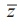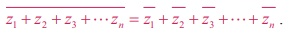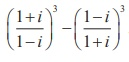Home | | Maths 12th Std | Conjugate of a Complex Number

# Conjugate of a Complex Number

In this section, we study about conjugate of a complex number, its geometric representation, and properties with suitable examples.

Conjugate of a Complex Number

In this section, we study about conjugate of a complex number, its geometric representation, and properties with suitable examples.

### Definition 2.3

The conjugate of the complex number x + iy is defined as the complex number x ŌłÆ i y.

The complex conjugate of z is denoted by. To get the conjugate of the complex number z , simply change i by ŌłÆi in z. For instance 2 ŌłÆ 5i is the conjugate of 2 + 5i. The product of a complex number with its conjugate is a real number.

For instance, (i) (x+iy)( x+iy) = x2 ŌĆō (iy) = x2+y2

(ii) (1 + 3i)(1-3i) = (1)2 ŌĆō (3i)2 = 1 + 9 =10.

Geometrically, the conjugate of z is obtained by reflecting z on the real axis.

## 1. Geometrical representation of conjugate of a complex numberTwo complex numbers x+iy and x-iy are conjugates to each other. The conjugate is useful in division of complex numbers. The complex number can be replaced with a real number in the denominator by multiplying the numerator and denominator by the conjugate of the denominator. This process is similar to rationalising the denominator to remove surds.

## 2. Properties of Complex ConjugatesLet us verify some of the properties.

Property

For any two complex numbers z1 and z2, we haveProof

Let z1 = x1 + iy1 , z2 = x2 + iy2 , and x1 , x2 , y1 , and y2 Ōłł RIt can be generalized by means of mathematical induction to sums involving any finite number of terms### Property### Proof

Let z1 = x1 + iy1 and z2 = x2 + iy2.

Then, z1z2   = ( x1 + iy1 )( x2 + iy2 ) = ( x1 x2 - y1 y2 ) + i ( x1 y2 + x2 y1 ) .### Property

A complex number z is purely imaginary if and only if z = ŌłÆ### Proof

Let z = x i + y . Then by definition= ŌłÆ iy

Therefore, z = ŌłÆŌćö x + iy = ŌłÆ (x ŌłÆ iy)

Ōćö 2x = 0 Ōćö  x = 0

Ōćö z is purely imaginary.

Similarly, we can verify the other properties of conjugate of complex numbers.

Example 2.3

Write 3+4i / 5-12i in the xi + y form, hence find its real and imaginary parts.

Solution

To find the real and imaginary parts of [3+4i] / [5-12i] , first it should be expressed in the rectangular form x + iy. To simplify the quotient of two complex numbers, multiply the numerator and denominator by the conjugate of the denominator to eliminate i in the denominator.This is in the x + iy form.

Hence real part is ŌĆō 33/169 and imaginary part is 56/169

Example 2.4

Simplifyinto rectangular form

Solution### Example 2.5

If, find the complex number z in the rectangular form

### SolutionExample 2.6

If z1 = 3 ŌłÆ 2i and z2 = 6  + 4i , find z1/z2 in the rectangular form

Solution

Using the given value for z1 and z2 the value of z1/z2 =### Example 2.7

Find zŌłÆ1, if z = (2+3i) (1ŌłÆ i).

### Solution

We have z = (2 + 3i )(1- i ) = (2 + 3) + (3 - 2)i = 5 + i

ŌćÆ  z-1 = 1/ z = 1/[5 + i ].

Multiplying the numerator and denominator by the conjugate of the denominator, we getExample 2.8SolutionTags : Definition, Geometrical representation, Properties, Proof, Solved Example Problems , 12th Mathematics : UNIT 2 : Complex Numbers
Study Material, Lecturing Notes, Assignment, Reference, Wiki description explanation, brief detail
12th Mathematics : UNIT 2 : Complex Numbers : Conjugate of a Complex Number | Definition, Geometrical representation, Properties, Proof, Solved Example Problems